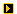YOU CAN CODE!With The Case Of UCanCode.net  Release The Power OF  Visual C++ ! Home |  Products | Purchase |  Support | DownloadsView in English
View in Japanese
View in
참고
View in Français
View in Italiano
View in 中文(繁體)Download EvaluationPricing & Purchase?E-XD++Visual C++/ MFC ProductsOverviewElectronic Form SolutionVisualization & HMI SolutionPower system HMI SolutionBar code labeling SolutionWorkflow SolutionCoal industry HMI SolutionInstrumentation Gauge SolutionReport Printing SolutionGraphical modeling SolutionGIS mapping solutionVisio graphics solutionIndustrial monitoring SolutionFlowchart and diagramming SolutionOrganization Diagram SolutionGraphic editor Source CodeUML drawing editor Source CodeMap Diagramming SolutionArchitectural Graphic Drawing SolutionRequest EvaluationActiveX COM ProductsOverviewTechnical Support# Get Ready to Unleash the Power of UCanCode .NET

UCanCode Software focuses on general application software development. We provide complete solution for developers. No matter you want to develop a simple database workflow application, or an large flow/diagram based system, our product will provide a complete solution for you. Our product had been used by hundreds of top companies around the world!

"100% source code provided! Free you from not daring to use components because of unable to master the key technology of components!"

# VC++ Article Draw Rotate / slant text strings SetWorldTransform

## Introduction

The function provided here can be used to draw a text string with an oblique or slant angle. Such text outputs are useful in isometric or perspective 3D views to make the text strings look like in their 3D space. An example is shown in the following picture:## Background

Windows GDI function ``` TextOut()``` does not allow a text slant angle. To draw such slanted strings, we need to set a transformation using ``` SetWorldTransform()```. Windows drawing function will then take care of the shearing and rotation of the output. This procedure is incorporated into a new function similar to Windows ``` TextOut() ``` function:

```void ObliqueTextOut( CDC *dc, int oblique, int x,int y,const CString &Text )
```

This function has the same arguments as Windows ``` TextOut()``` function with an additional argument, `oblique`, to specify the text slant angle. The function can be placed where ``` TextOut()``` is normally used.

## Using the code

Insert the function source code into your source code file. Call the function at places where you would normally call Windows ``` TextOut()``` function. Remember to select the font, set the text background mode, color and background color etc, as you would normally do before calling ``` TextOut()```.

Angle ``` oblique``` is positive if the text slants forward(to the right) and negative if it slants backwards(to the left). The ``` oblique ``` angle, `s`, in the figure below is positive. The angle is in 1/10th degrees. Therefore, if the text slants forward 15 degrees, oblique=150.

## Points of Interest

The key to the question is to set up the transformation in DC. Function ``` SetWorldTransform() ``` needs an ``` XFORM``` structure for the transformation. Therefore, we need to prepare the ``` XFORM``` structure before calling ``` SetWorldTransform( )```. `XFORM` has 6 member data. They are ``` eM11```, `eM21`, `eM12`, `eM22`, `eDx`, `eDx`. They are defined as:

```X = eM11 * x + eM21 * y + eDx
Y = eM12 * x + 2M22 * y + eDy```

where ``` (x,y) ```are the World coordinates and ``` (X,Y)``` are the Paper space coordinates.

In the figure below, ``` x,y``` are the World space axes. The string will always be drawing at ``` (0,0)``` and horizontally in the world space. ```xs,ys ``` are the Sheared space axes. The transformation from World to the Sheared space is:

```xs = x - y * tan(s)
ys = y```

where ``` s``` is the slant or oblique angle.The Paper space is noted as ``` X,Y```. From the Sheared space to Paper space, the transformation is a rotation(angle ``` r```) and translation`(Xo,Yo)`.

```X = Xo + xs * cos(r) + ys * sin(r)
Y = Yo + ys * cos(r) - xs * sin(r)```

Where ``` (Xo,Yo)``` are simply the text insertion point in Paper space. Substitute ``` (xs,ys)``` into the above, we get:

```X = cos(r) * x + (sin(r)-tan(s)*cos(r)) * y + Xo
Y = -sin(r) * x + (cos(r)+tan(s)*sin(r)) * y + Yo
```

Compare this to the ```XFORM ``` structure, it is obvious that:

```eM11 = cos(r)
eM21 = sin(r) - tan(s) * cos(r)
eM12 = -sin(r)
eM22 = cos(r) + tan(s) * sin(r)
eDx = Xo
eDy = Yo```

The above is translated into function code(```dc ``` is the input device context):

```XFORM xForm;
xForm.eDx = (float) x;
xForm.eDy = (float) y;
xForm.eM11 = (float) cos(txtRotate);
xForm.eM21 = (float) (sin(txtRotate) - tan(txtOblique)*cos(txtRotate));
xForm.eM12 = (float) -sin(txtRotate);
xForm.eM22 = (float) (cos(txtRotate) + tan(txtOblique)*sin(txtRotate));
SetWorldTransform( dc->m_hDC, &xForm );```

The call to ``` SetGraphicsMode() ```is needed. Otherwise, function ``` SetWorldTranform() ```will have no effect. Since now we are drawing in World space, we need to adjust the font's rotation(`lfEscapement`) to be horizontal and the character orientation(`lfOrintation`) to be from the World X-axis.

```LOGFONT lgf;
dc->GetCurrentFont()->GetLogFont( &lgf );
...
lgf.lfOrientation -= lgf.lfEscapement;
lgf.lfEscapement = 0;
CFont horFont;
horFont.CreateFontIndirect( &lgf );
CFont *OldFont = dc->SelectObject( &horFont );```

Now, we can call:

`dc->TextOut( 0,0, Text );`

The work is done. But before returning, we need to restore the graphics mode and font:

```ModifyWorldTransform( dc->m_hDC, &xForm, MWT_IDENTITY );
SetGraphicsMode( dc->m_hDC, GM_COMPATIBLE );
dc->SelectObject( OldFont );```